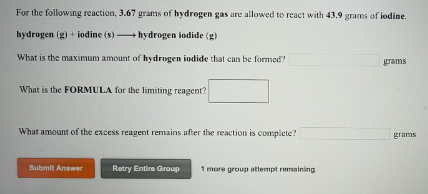# Problem: For the following reaction, 3.67 grams of hydrogen gas are allowed to react with 43.9 grams of iodine. hydrogen (g) + iodine (s) → hydrogen iodide (g)What is the maximum amount of hydrogen iodide that can be formed?What is the FORMULA for the limiting reagent? What amount of the excess reagent remains after the reaction is complete?

###### FREE Expert Solution
94% (105 ratings)###### Problem Details

For the following reaction, 3.67 grams of hydrogen gas are allowed to react with 43.9 grams of iodine.

hydrogen (g) + iodine (s) → hydrogen iodide (g)

What is the maximum amount of hydrogen iodide that can be formed?

What is the FORMULA for the limiting reagent?

What amount of the excess reagent remains after the reaction is complete?